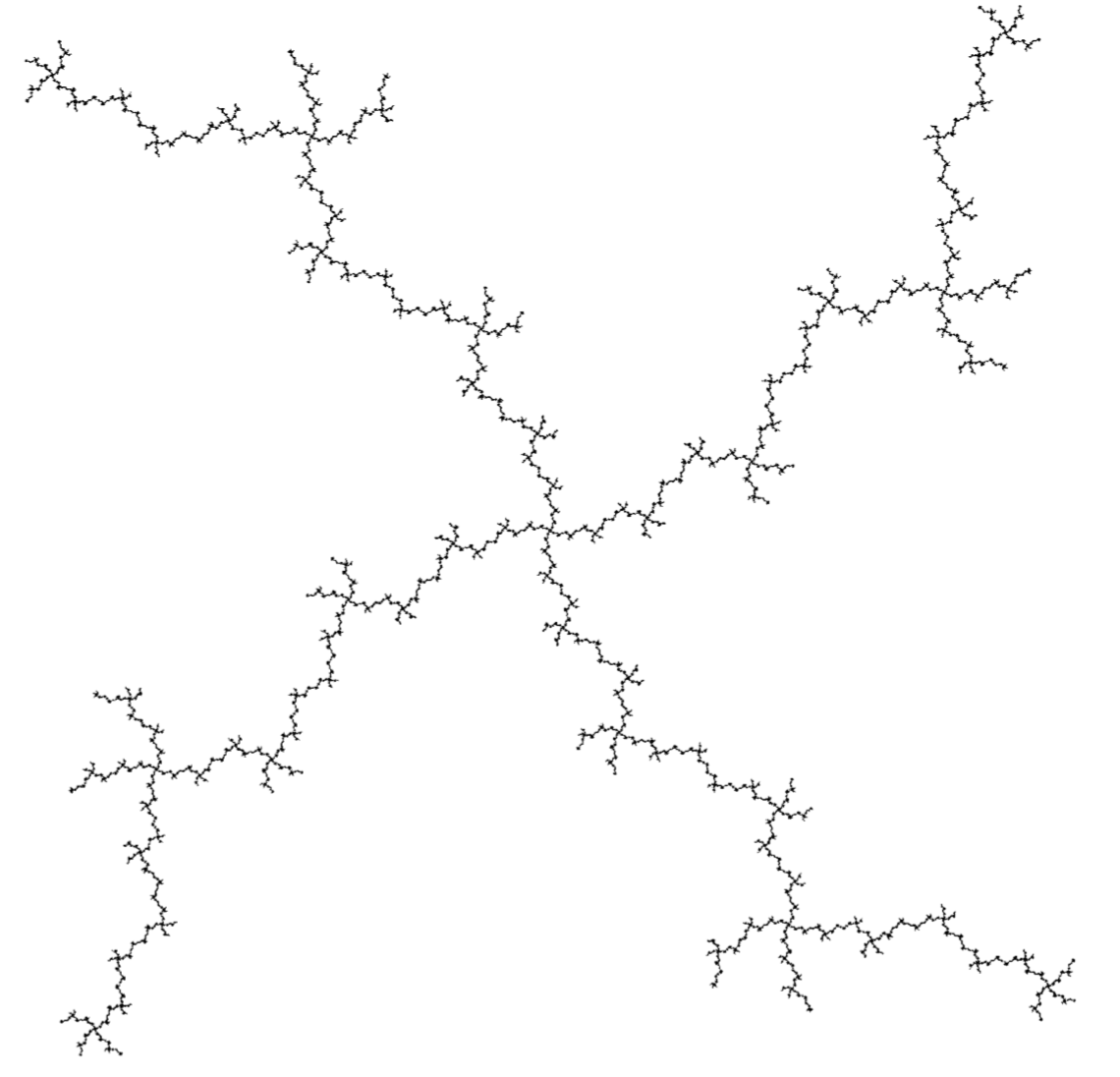# Tag: Zvonkin

A Belyi-extender (or dessinflateur) is a rational function $q(t) = \frac{f(t)}{g(t)} \in \mathbb{Q}(t)$ that defines a map
$q : \mathbb{P}^1_{\mathbb{C}} \rightarrow \mathbb{P}^1_{\mathbb{C}}$
unramified outside $\{ 0,1,\infty \}$, and has the property that $q(\{ 0,1,\infty \}) \subseteq \{ 0,1,\infty \}$.

An example of such a Belyi-extender is the power map $q(t)=t^n$, which is totally ramified in $0$ and $\infty$ and we clearly have that $q(0)=0,~q(1)=1$ and $q(\infty)=\infty$.

The composition of two Belyi-extenders is again an extender, and we get a rather mysterious monoid $\mathcal{E}$ of all Belyi-extenders.

Very little seems to be known about this monoid. Its units form the symmetric group $S_3$ which is the automrphism group of $\mathbb{P}^1_{\mathbb{C}} – \{ 0,1,\infty \}$, and mapping an extender $q$ to its degree gives a monoid map $\mathcal{E} \rightarrow \mathbb{N}_+^{\times}$ to the multiplicative monoid of positive natural numbers.

If one relaxes the condition of $q(t) \in \mathbb{Q}(t)$ to being defined over its algebraic closure $\overline{\mathbb{Q}}$, then such maps/functions have been known for some time under the name of dynamical Belyi-functions, for example in Zvonkin’s Belyi Functions: Examples, Properties, and Applications (section 6).

Here, one is interested in the complex dynamical system of iterations of $q$, that is, the limit-behaviour of the orbits
$\{ z,q(z),q^2(z),q^3(z),… \}$
for all complex numbers $z \in \mathbb{C}$.

In general, the 2-sphere $\mathbb{P}^1_{\mathbb{C}} = S^2$ has a finite number of open sets (the Fatou domains) where the limit behaviour of the series is similar, and the union of these open sets is dense in $S^2$. The complement of the Fatou domains is the Julia set of the function, of which we might expect a nice fractal picture.

Let’s take again the power map $q(t)=t^n$. For a complex number $z$ lying outside the unit disc, the series $\{ z,z^n,z^{2n},… \}$ has limit point $\infty$ and for those lying inside the unit circle, this limit is $0$. So, here we have two Fatou domains (interior and exterior of the unit circle) and the Julia set of the power map is the (boring?) unit circle.

Fortunately, there are indeed dynamical Belyi-maps having a more pleasant looking Julia set, such as this oneBut then, many dynamical Belyi-maps (and Belyi-extenders) are systems of an entirely different nature, they are completely chaotic, meaning that their Julia set is the whole $2$-sphere! Nowhere do we find an open region where points share the same limit behaviour… (the butterfly effect).

There’s a nice sufficient condition for chaotic behaviour, due to Dennis Sullivan, which is pretty easy to check for dynamical Belyi-maps.

A periodic point for $q(t)$ is a point $p \in S^2 = \mathbb{P}^1_{\mathbb{C}}$ such that $p = q^m(p)$ for some $m > 1$. A critical point is one such that either $q(p) = \infty$ or $q'(p)=0$.

Sullivan’s result is that $q(t)$ is completely chaotic when all its critical points $p$ become eventually periodic, that is some $q^k(p)$ is periodic, but $p$ itself is not periodic.

For a Belyi-map $q(t)$ the critical points are either comlex numbers mapping to $\infty$ or the inverse images of $0$ or $1$ (that is, the black or white dots in the dessin of $q(t)$) which are not leaf-vertices of the dessin.

Let’s do an example, already used by Sullivan himself:
$q(t) = (\frac{t-2}{t})^2$
This is a Belyi-function, and in fact a Belyi-extender as it is defined over $\mathbb{Q}$ and we have that $q(0)=\infty$, $q(1)=1$ and $q(\infty)=1$. The corresponding dessin is (inverse images of $\infty$ are marked with an $\ast$)The critical points $0$ and $2$ are not periodic, but they become eventually periodic:

$2 \rightarrow^q 0 \rightarrow^q \infty \rightarrow^q 1 \rightarrow^q 1$
and $1$ is periodic.

For a general Belyi-extender $q$, we have that the image under $q$ of any critical point is among $\{ 0,1,\infty \}$ and because we demand that $q(\{ 0,1,\infty \}) \subseteq \{ 0,1,\infty \}$, every critical point of $q$ eventually becomes periodic.

If we want to avoid the corresponding dynamical system to be completely chaotic, we have to ensure that one of the periodic points among $\{ 0,1,\infty \}$ (and there is at least one of those) must be critical.

Let’s consider the very special Belyi-extenders $q$ having the additional property that $q(0)=0$, $q(1)=1$ and $q(\infty)=\infty$, then all three of them are periodic.

So, the system is always completely chaotic unless the black dot at $0$ is not a leaf-vertex of the dessin, or the white dot at $1$ is not a leaf-vertex, or the degree of the region determined by the starred $\infty$ is at least two.

Going back to the mystery Manin-Marcolli sub-monoid of $\mathcal{E}$, it might explain why it is a good idea to restrict to very special Belyi-extenders having associated dessin a $2$-coloured tree, for then the periodic point $\infty$ is critical (the degree of the outside region is at least two), and therefore the conditions of Sullivan’s theorem are not satisfied. So, these Belyi-extenders do not necessarily have to be completely chaotic. (tbc)